# Lasionectrin Structure Elucidation

### Lasionectrin

Here we will consider the elucidation of a structure which is of modest size and complicity and does not contain any “exotic” substructures. However this problem allows us to discuss some important issues that can influence the results of computer-assisted structure elucidation.

El Aouad at al1 isolated a new naphthopyrone (structure 1) named Lasionectrin with antiplasmodial properties from fermentation broths of a Lasionectria species. The article constitutes the first account on the isolation of a natural product from fungi of this genus.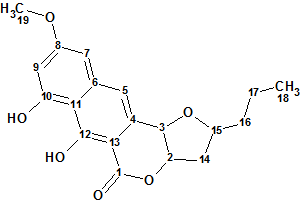Structure 1

A pseudomolecular ion at m/z 345.1335 by (+)-ESI-TOFMS and the presence of 19 signals in the 13C NMR spectrum determined a molecular formula of C19H20O6 for compound 1. The UV spectrum displayed absorptions at 216, 260, 306, and 363 nm, characteristic of a 3,4-dihydro-9,10-dihydroxy-7-methoxynaphtho[2,3-c]pyran-1-one moiety (naphthopyrone, below), and similar to those observed in the structurally related compounds.Compound 1

The authors1 used this substructure as a starting point to elucidate the full structure of the new compound. The latter was achieved by application of 1D and 2D NMR data.

We will consider the compound under investigation as a real “unknown” and will try to solve the problem “ab initio“. 1D NMR spectra along with 42 HMBC and 8 COSY correlations derived by authors1 from 2D NMR data were input into Structure Elucidator (Table 1).
Table 1.

 C Label δC CHn δH t Multp COSY HMBC C 1 171.2 C C 2 84.2 CH 5.24 u 2.58, 4.93 75.00, 80.40 C 3 75 CH 4.93 d(1.6) 5.24 120.70, 131.90, 98.50, 84.20,163.6 C 4 131.9 C C 5 120.7 CH 7.23 s 98.50, 171.20, 75.00, 101.10, 110.30, 142.10, 163.60 C 6 142.1 C C 7 101.1 CH 6.8 d(2.1) 6.51 164.40, 120.70, 103.50, 110.30 C 8 164.4 C C 9 103.5 CH 6.51 d(2.1) 6.8 164.40, 159.50, 101.10, 110.30 C 10 159.5 C C 11 110.3 C C 12 163.6 C C 13 98.5 C C 14 40.8 CH2 2.12 u 80.40, 39.40 C 14 40.8 CH2 2.58 u 5.24, 4.33 75.00, 84.20, 39.40 C 15 80.4 CH 4.33 u 2.58, 1.70 40.80, 20.30, 75.00, 131.9 C 16 39.4 CH2 1.57 u C 16 39.4 CH2 1.7 u 4.33, 1.49 20.30, 80.40, 40.80, 14.40 C 17 20.3 CH2 1.49 u 1.7 39.40, 80.40, 14.40 C 17 20.3 CH2 1.39 u 0.97 C 18 14.4 CH3 0.97 t(7.3) 1.39 20.30, 39.40 C 19 56 CH3 3.89 s 164.4

Using these data a Molecular Connectivity Diagram (MCD) was created (Figure
1).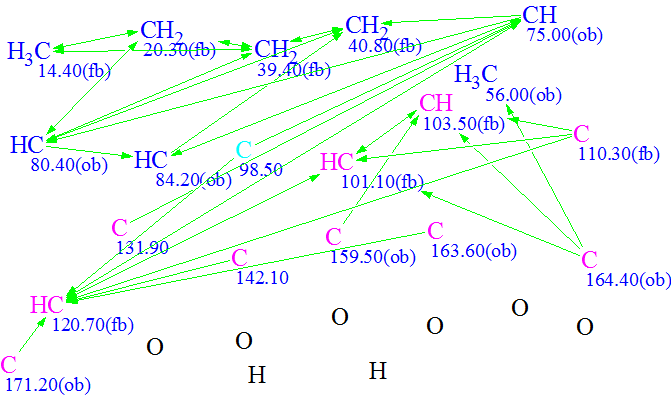Figure 1. Molecular Connectivity Diagram

The atom properties displayed in the MCD were assigned by the program, and also partly by the user considering the molecular composition (particularly, absence of nitrogen atoms in the molecular formula) and characteristic chemical shifts2. Hybridization of C(98.5) was set by the program as “sp2 or sp3” to take in account possibility of carbon double bonds or O-C-O fragments in the structure.

One of the specific features of Structure Elucidator software is that it is capable of taking into account signal multiplicities in 1H NMR spectra. A signal multiplicity M=n+1 in a first order spectrum allows the determination of the total number n of hydrogen atoms attached to carbons neighboring a carbon with which the signaling hydrogen is connected. If n values are reliably determined for some atoms, the user can add corresponding numbers to the property description of carbon atoms. As we will see later, introduction of these numbers gives significant additional constraints to the program during the structure generation, which results in accelerating the process and reducing number of possible structures. Therefore application of 1H signal multiplicities is very attractive during the computer-assisted structure elucidation.

Let us use this example to show how numbers of hydrogen atoms are input into the program. Table 1 shows that CH3 (C-18) produces a triplet with J=7.3 Hz at 0.97 ppm and hence the number of hydrogen atoms in the first sphere of atom C-18’s environment is expected to be 2. This number is typed in the “Number of hydrogens on neighbor atoms” field (Figure 2).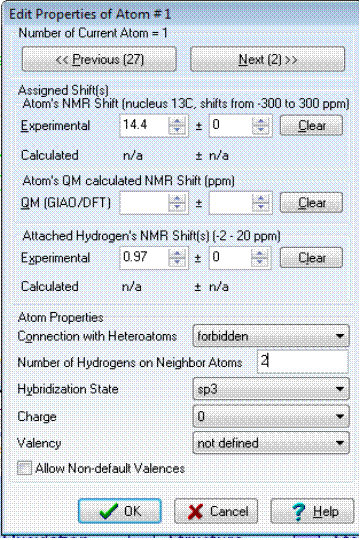Figure 2. Structure Elucidator window intended for setting and editing atom properties.

It should be noted that the procedure of setting n values is very delicate and should be performed with great caution. In fact, when assigning an n number to some carbon atom we introduce a new “axiom” and if it turns out that the axiom is false we will never obtain a valid solution. To make a decision, the values of JHH coupling constants are usually analyzed. If JHH<2.5 Hz, it is usually assumed that a signal is split due to a long-range coupling and the corresponding n value is equal to zero. Experience shows that the soundest approach is to use legible and crisp multiplets (for instance, singlets, doublets, and triplets of methyl groups).

With the data presented in Table 1, the following n values were introduced (Table 2):

Table 2. Numbers of hydrogen atoms on neighboring carbon atoms.

 Label of C atom C3 C5 C7 C9 C18 C19 δH 4.93 7.23 6.80 6.51 0.97 56.0 n 0 0 0 0 2 0

Let us remember that here we are modeling a situation when we know nothing about the real structure of the molecule under investigation and we can not guess that in structure 1 the coupling constant JHH=1.6 Hz (see Table 1, line related to C3 and structure 1) corresponds to 3i interaction of vicinal protons.

Checking MCD for contradictions was performed and as a result the program detected the presence of nonstandard correlations, NSCs. Because the structure and shift assignment were known from the work1, checking of the “proposed” 1 structure by 2D NMR data allowed us to establish 5 NSCs (nJCH, n>3) in HMBC and one NSC (nJHH, n>3) in the COSY spectrum. The nonstandard correlations are marked by red lines in structure 1a, where the connectivity C7-C9 belongs to COSY spectrum.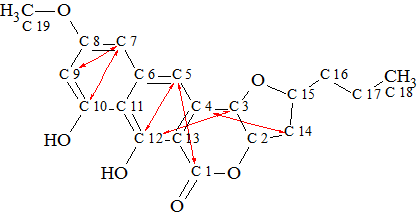1a

The most practical strategy of overcoming contradictions (the presence of NSCs in 2D NMR data) is using Fuzzy Structure Generation (FSG)3. Therefore FSG was initiated with the following parameters: m=0–20, a=16, “Stop generation when structure generated”, “Calculate carbon spectra during generation”, “Reject structures with d>4 ppm and d(max)>20 ppm”.

These options mean that the program will try different m values (number of NSCs) under conditions that the number of NSCS and true lengths of each NSC are unknown. If the process reaches m=mg (when at least one structure will be stored as a result of structure generation) the generation process is completed and the generated structures are checked by 13C NMR spectrum prediction. Generated structures with big deviations (d>4 ppm) are rejected during the structure filtering.

The following FSG runs were performed:

Run 1. Generated structures were stored only at mg=5. Results: k=74143 → 4, tg=6m 20s. All structures are shown in Figure 3.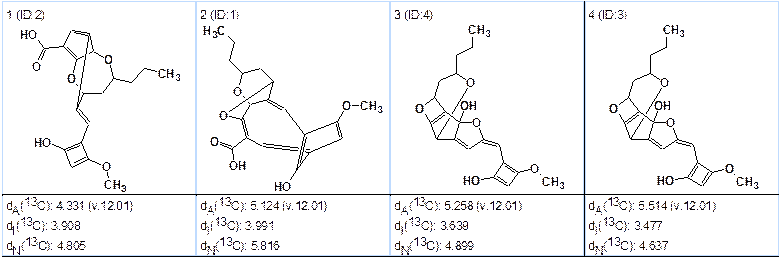Figure 3. Resulted structures obtained from the first program run.

Large deviations and “exotic” structures indicate a wrong solution was obtained. Therefore it is necessary to repeat structure generation with m=6.

Run 2. The generation option m=6 was set. Results: k=1,783,619 → 158 → 94, tg=3h 2m. The correct structure was not generated. Four “best” structures of the ranked file are shown in Figure 4.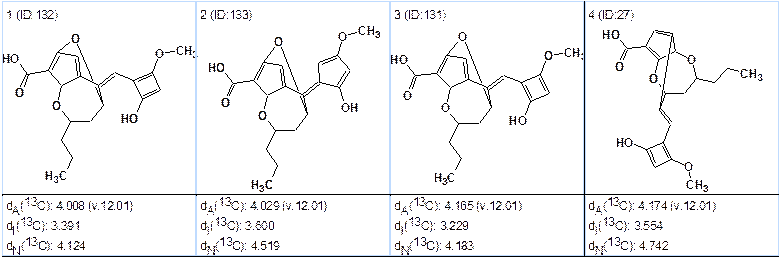Figure 4. Top structures of the file obtained from the second program run.

We see again that the solution found is not valid, which should be expected because we erroneously set the number of hydrogen atoms equal to zero in the first sphere of carbon C3.

The performed computational experiments have shown the that initial data used contain an incorrect assumption (false “axiom”). Looking for the false axiom is usually started by removing such user constraints that can lead to loss of correct structure. In the current situation the most suspicious is the constraint n(H)=0 introduced for sp3 carbon C(75.00) because other similar constraints (n-values) are related to methyl groups and to protons which are supposed to be aromatic. The n(H)=0 constraint was removed on the MCD at C(75.00) and the FSG was repeated under the same conditions.

Run 1a. Two “exotic” molecules were stored at mg=4 (tg=1m 23s), both with large calculated deviations.

Run 2a. At m=5 the result was: k=337637 → 47 → 28, tg=51m and all structures (rather exotic) and having large deviations (4–5.5ppm) were again rejected.

Run 3a. Generation was performed with m=6. Results: k=6,680,220 → 822 → 385, tg=19h 30m.

The top 6 structures of the file ranked by deviations dA (calculated using HOSE approach) are shown in Figure 5.Figure 5. Top structures obtained from the program run 3a.

The best structure #1 coincides with structure 1 and the average deviations are acceptable. Values of maximum deviations calculated by all three methods (HOSE, Increments, and Neural Nets) support the selection. Hence the valid solution was found with the presence of 6 nonstandard 2D NMR correlations of unknown length. It is worthy to note that after removing the single erroneous constraint the number of structures generated at m=6 became four times more while the tg value became six times longer. This is the trade-off for getting a valid solution in almost automatic mode at the presence of six NSCs.

Now when the correct structure is determined it would be interesting to understand the cause of such a small coupling constant (1.5 Hz) which provoked us to introduce a wrong assumption. As was mentioned, an erroneous number n=0 was ascribed to CH(75.00, 4.93) because a doublet with JHH=1.5 was observed at δH=4.93ppm. The small value of the coupling constant played the role of a pitfall in this case. It is known4 that a vicinal coupling constant depends on a series of factors (dihedral angles, valence angles, substituent effect, etc.). We can expect that quantum chemical calculations would shed light on a real cause of the small coupling between vicinal atoms H3 and H4 which are cis-oriented (found in1 from NOESY spectrum). We can only draw attention to the fact that electronegative ethane substitutes reduce the value of vicinal interaction4. In structure 1, both atoms H3 and H4 have electronegative substitutes (oxygen atoms) as neighbors, which probably made a significant contribution to reducing the coupling constant. With the aid of ACD/CNMR Predictor we calculated coupling constants for H(4.93) using two models of the molecule: with and without stereobonds drawn to hydrogens from atoms C3 and C2. It came out that 3JHH=7 Hz when stereobonds are neglected and 3JHH=3.3 Hz when stereochemistry was taken into account. Unfortunately, these additional data can be obtained only posteriori, when the structure and its stereochemistry became known.

This example shows once more how the researcher should be careful when introducing constraints on the basis of multiplicities observed in the 1H NMR spectrum. Nonstandard correlations appear in 2D NMR data because peak intensities corresponding to correlations of different lengths can be of the same volume. To understand reasons of NSCs including into 2D NMR data of structure 1, we inspected the HMBC and COSY spectra presented in the Supporting Information related to article1. Inspection showed that intensity of 4JHH COSY peak (6.8–6.51, in square) indeed is of the order which is intrinsic for other “standard” COSY peaks (see Figure 6). However inspection of the HMBC spectrum (Figure 7) allowed us to reveal that three NSC peaks (6.8 to 159.5, 4.93 to 163.5, and 2.58 to 131.9) are absent from experimental data at all, therefore they were completely imaginary.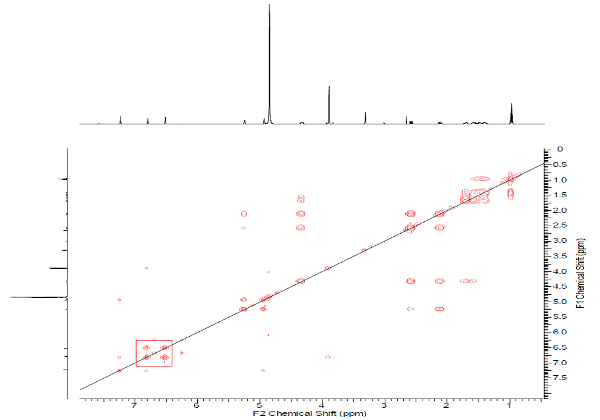Figure 6. COSY spectrum of Lasionectrin.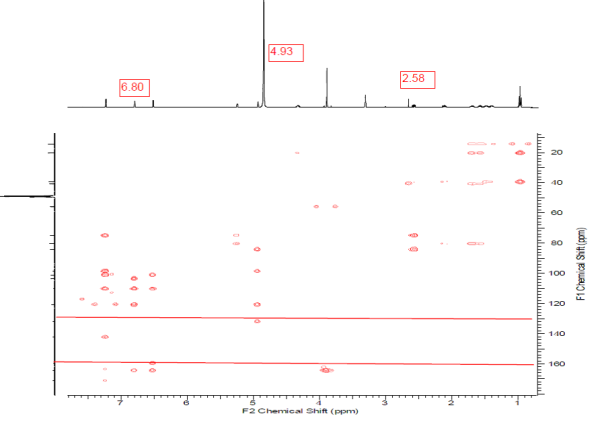Figure 7. HMBC spectrum of Lasionectrin.

Formally speaking, the three mentioned absentee peaks that were included into HMBC data are carriers of false structural information, but the program demonstrated the capability of finding a valid solution to the problem even when the initial information was false. As was mentioned, the trade-off for getting a valid solution from the false information is a much longer time of fuzzy structure generation.

For sake of completeness we excluded three false HMBC correlations and repeated solution with the true experimental data containing only 3 NSCs—two in HMBC and one in COSY.

Run 1b. All numbers of hydrogens at neighbor carbons were removed on the MCD. Parameters of FSG: mg=3, a=16, 13C calculation is performed during the structure generation.

Results: k=192,279 → 19 →9, tg=46m. The correct structure was selected.

Run 2b. Numbers of hydrogens at neighbor carbons were restored as shown in Table 2 except of atom C3. At the same FSG options as in Run 1b we obtained the following results:

k=21,837 → 10 → 6, tg=7m 11s. The top of ranked output file is shown in Figure 8.Figure 8. Top structures obtained from the program Run 2b.

We see that ranking structures with dA(13C) deviations, supported by values of maximal deviations, again allows us to select the best structure which coincides with structure 1.

Comparison of the results obtained from the two last runs convincingly demonstrates the advantages of employing multiplicities determined in the 1H NMR spectra: the number of generated structures is almost ten times smaller and the time of structure generation is 6 times smaller when multiplicities are used.

In the end we must once more underline that the application of 1H multiplicities requires one to be very careful. Constraints based on multiplicities of methyl groups seem be the most reliable.

### References

1. N. El Aouad, G. Pérez-Moreno, P. Sánchez, J. Cantizani, F. J. Ortiz-López, J. Martín, V. González-Menéndez, L.M. Ruiz-Pérez, D. González-Pacanowska, F. Vicente, G. Bills, F. Reyes. Lasionectrin, a Naphthopyrone from a Lasionectria sp. J. Nat. Prod., 75 (6):1228–1230, 2012.
2. Pretsch, E.; Clerc, T.; Seibl, J.; Simon, W., Tables of Spectral Data for Structure Determination of Organic Compounds. Springer-Verlag: Berlin, 1989.
3. M.E. Elyashberg, A.J. Williams, Blinov K.A. Contemporary Computer-Assisted Approaches to Molecular Structure Elucidation, Cambridge, RSC Publishing, 2012.
4. Günther, H., NMR Spectroscopy. Wiley: Chichester, 2001.